# 车辆轨迹异常行为研究 Research on Abnormal Trajectory Behavior of Vehicles

DOI: 10.12677/HJDM.2019.93014, PDF, HTML, XML, 下载: 597  浏览: 1,594

Abstract: In this paper, an outlier detection model is established. After removing data redundancy, the outlier data is cleaned up, that is partial selective removal of trajectory data drift. Then a map matching algorithm based on Hidden Markov Model and real-time electronic map are used to match a segment of the trajectory offset to the nearest road network, which completes the matching of the transportation road map of the transport vehicle and corrects the abnormal section of the vehicle trajectory better.

1. 研究背景

2. 问题分析

3. 建立模型

3.1. 轨迹数据预处理

3.2. 去除数据冗余

3.3. 样条插值

$\Delta :a={x}_{0}<{x}_{1}<\cdots <{x}_{n}=b$

3.4. 噪点过滤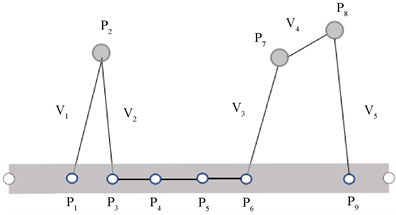Figure 1. Schematic diagram of path noise

1) 均值过滤。如果某一个异常点对于它前后两个观测点位置偏移量极大时，可以采用均值过滤的方法，使车辆在单位时间内的位移距离更加合理。第K个点的位置估值可以表示为：

${P}_{K}=\frac{{P}_{k-1}+{P}_{k+1}}{2}$

2) 卡尔曼滤波过滤。卡尔曼过滤根据测量值和运动趋势得到轨迹估值，卡尔曼过滤基于轨迹符合线性模型的假设，根据运动物体转移概率矩阵的关系，对异常值的采集点预测并行校正  。

$X\left(k+1\right)=A\left(k\right)X\left(k\right)+T\left(k\right)W\left(k\right)$

$Z\left(k\right)=H\left(k\right)X\left(k\right)+V\left(k\right)$

$M=E\left[\left({x}_{i}-{{x}^{\prime }}_{i}\right){\left({x}_{i}-{{x}^{\prime }}_{i}\right)}^{\text{T}}\right]$

$\begin{array}{l}X\left(k+1,k\right)=A\left(k\right)X\left(k,k\right)\\ Z\left(k+1,k\right)=H\left(k\right)X\left(k+1,k\right)\end{array}$

3) 基于启发式的异常检测模型。

${V}_{K}=\frac{S}{t}$

${V}_{K}$ 明显大于某个阈值即高于200 km/h时，认为为异常点。

3.5. 基于隐马尔科夫的地图匹配算法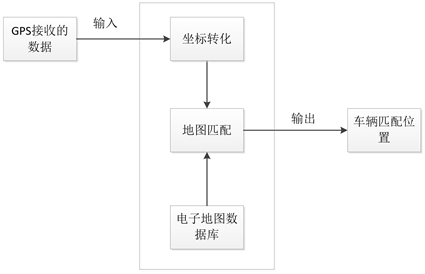Figure 2. Map matching principle

Step 1：确定参数：

HMM的5个基本因素，由状态变量数，观测变量数，状态转移矩阵，观测概率矩阵，初始状态概率向量组成  。

1) 确定观察变量。从车联网采集的数据中得到的位置信息(包括经度，纬度)；

2) 隐常状态。此时在路网上的运输车的实际所在位置；

3) 观测概率。观测经纬度离旁边道路近，那么真实点在这个路段的概率越大  ，假设服从高斯分布设有：

$p\left({z}_{t}|{r}_{i}\right)=\frac{1}{\sqrt{2\text{π}{\sigma }_{z}}}{\text{e}}^{-0.5{\left(\frac{‖{z}_{t}-{x}_{t,i}‖}{{\sigma }_{z}}\right)}^{2}}$

${\sigma }_{z}=1.4826media{n}_{t}\left(‖{z}_{t}-{x}_{t,i}‖\right)$

4) 状态转移概率。在两个时间点候选路段的转换概率：

$p\left({d}_{t}\right)=\frac{1}{\beta }{\text{e}}^{-{d}_{t}/\beta }$

${d}_{t}=|‖{z}_{t}-{z}_{t+1}‖-‖{x}_{t,i}-{x}_{t+1,i}‖|$$\beta =\frac{1}{\mathrm{ln}\left(2\right)}media{n}_{t}\left(dt\right)$

5) 状态转移矩阵A：

${a}_{ij}=P\left({q}_{t+1}={r}_{j}|{q}_{t}={r}_{i}\right)={\text{e}}^{-{r}_{ij}}$

${r}_{j}$ 是时刻t + 1时所在路段， ${r}_{i}$ 是时刻t所在路段， ${r}_{ij}$ 有三种状态，当 ${r}_{ij}$ 为1时，相邻， ${r}_{ij}$ 为为无穷大时，不相邻， ${r}_{ij}$ 等于0时，在同一路段。

Step 2：Viterbi算法求解

$\begin{array}{l}{\delta }_{t}\left(i\right)=\mathrm{max}P\left({i}_{t}=i,{i}_{t-1},\cdots ,{i}_{1},{o}_{t},\cdots ,{o}_{1}|\lambda \right)\\ {\delta }_{t+1}\left(i\right)={\mathrm{max}}_{{i}_{1},\cdots ,{i}_{t}}P\left({i}_{t}=i,{i}_{t-1},\cdots ,{i}_{1},{o}_{t},\cdots ,{o}_{1}|\lambda \right)\end{array}$

${\psi }_{t}\left(i\right)=\mathrm{arg}\mathrm{max}\left[{\delta }_{t-1}\left(j\right){a}_{ij}\right]$

4. 实验求解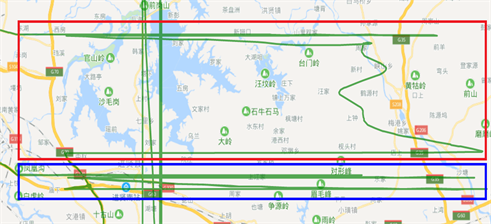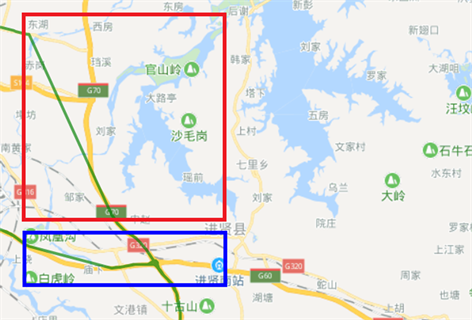Figure 4. Removing the anomaly data graph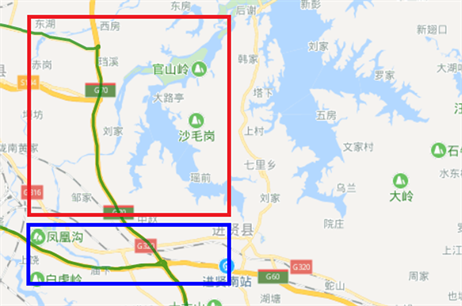Figure 5. Map matching algorithm after processing

5. 总结

  张永鹏, 甘庆华. 物联网下的智慧交通大数据挖掘系统探析[J]. 数字技术与应用, 2018, 36(4): 191-192.  范恒飞. 基于车联网的车辆运动轨迹追踪技术研究与实现[D]: [硕士学位论文]. 北京: 北京邮电大学, 2017.  孙川, 吴超仲, 褚端峰, 杜志刚, 田飞. 基于车联网数据挖掘的营运车辆驾驶速度行为聚类研究[J]. 交通运输系统工程与信息, 2015, 15(6): 82-87.  燕荣杰, 王国庆, 戴汝泉, 钟勤俭, 张竹林. 车联网数据预处理[J]. 物联网技术, 2017, 7(1): 81-82+85.  张迎亚. 基于隐马尔可夫模型的车辆轨迹预测算法的研究[D]: [硕士学位论文]. 南京: 南京邮电大学, 2017.  乔少杰, 韩楠, 朱新文, 舒红平, 郑皎凌, 元昌安. 基于卡尔曼滤波的动态轨迹预测算法[J]. 电子学报, 2018, 46(2): 418-423.  王潇. 基于车联网数据挖掘的货运车辆驾驶行为聚类研究[J]. 无线互联科技, 2017(11): 113-114+130.